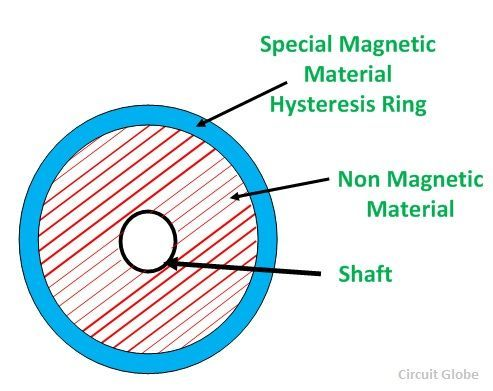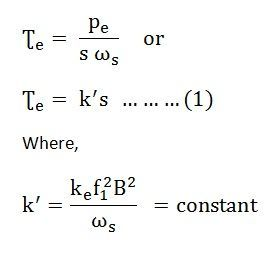# Hysteresis Motor

A Hysteresis Motor is a synchronous motor with a uniform air gap and without DC excitation. It operates both in single and three-phase supply. The torque in a Hysteresis Motor is produced due to hysteresis and eddy current induced in the rotor by the action of the rotating flux of the stator windings.

Contents:

The working of the motor depends on the working of the continuously revolving magnetic flux. For the split-phase operation, the stator winding of the motor has two single-phase supplies. This stator winding remains continuously connected to the single-phase supply both at the starting as well as the running of the motor.

The rotor of the motor is made up of a smooth chrome steel cylinder and it has no winding. It has high retentivity and because of this, it is very difficult to change the magnetic polarities once they are caused by the revolving flux of the rotor. The rotor of the hysteresis motor moves synchronously because the pole of the motor magnetically locks with the stator which has opposite polarities.

### Construction of Stator of Hysteresis Motor

The stator of the hysteresis motor produces a rotating magnetic field and is almost similar to the stator of the induction motor. Thus, the stator of the motor is connected either to a single supply or to the three-phase supply. The three-phase motor produces a more uniform rotating field as compared to that of the single-phase supply.
The stator winding of the single-phase hysteresis motor is made of permanent split capacitor type or shaded pole type. The capacitor is used with an auxiliary winding in order to produce a uniform field.

### Construction of Rotor of Hysteresis Motor

The rotor of the hysteresis motor consists of the core of aluminum or some other non-magnetic material that carries a layer of special magnetic material. The figure below shows the rotor of the hysteresis motor:The outer layer has a number of thin rings forming a laminated rotor. The rotor of the motor is a smooth cylinder, and it does not carry any windings. The ring is made of hard chrome or cobalt steel having a large hysteresis loop as shown in the figure below:

## Operation of a Hysteresis Motor

The following illustration shows the basic functioning of a hysteresis motor:When supply is given to the stator, a rotating magnetic field is produced. This magnetic field magnetizes the rotor ring and induces a pole within it. Due to the hysteresis loss in the rotor, the induced rotor flux lags behind the rotating stator flux. The angle δ between the stator magnetic field BS and the rotor magnetic field BR is responsible for the production of the torque. The angle δ depends on the shape of the hysteresis loop and not on the frequency.

Thus, the value of Coercive force and residual flux density of the magnetic material should be large. The ideal material would have a rectangular hysteresis loop as shown by loop 1 in the hysteresis loop figure. The stator magnetic field produces Eddy currents in the rotor. As a result, they produce their own magnetic field.

The eddy current loss is given by the equation shown below:Where,

• ke is a constant,
• f2 is the eddy current frequency,
• B is the flux density,

Where, s is the slip and f1 is the frequency of the stator.

The torque is given by the equation shown below:Now, the torque due to hysteresis loss is given by the equation shown below:The torque due to hysteresis is given as:From equation (1) it is clear that the torque is proportional to the slip. Therefore, as the speed of the rotor increases the value of Ʈe decreases. As the speed of the motor reaches synchronous speed, the slip becomes zero and torque also becomes zero.

As the electromagnet torque is developed by the motor is because of the hysteresis loss and remains constant at all rotor speeds until the breakdown torque. At the synchronous speed, the eddy current torque is zero and only torque due to hysteresis loss is present.

## Torque Speed characteristic of Hysteresis Motor

The speed torque curve of the motor is shown below:Here, curve 1 is the ideal curve, and curve 2 is the practical hysteresis motor curve. The torque-speed characteristic of the hysteresis motor is different from an induction motor. Since, at the synchronous speed, the torque developed by an induction motor becomes zero, whereas in the hysteresis motor, the torque is constant at all the speed even at the synchronous speed. Thus, from the curve, it is seen that the locked rotor, starting and pull out torque is equal.

The noise level of the hysteresis motor is very low as compared to the induction motor because it operates at a constant speed and its rotor is smooth. This type of motor is the smoothest running, quietest single phase motor, and is used for quality sound reproduction equipment like record players, tape recorders, etc. It is also employed in electric clocks and other timing devices.